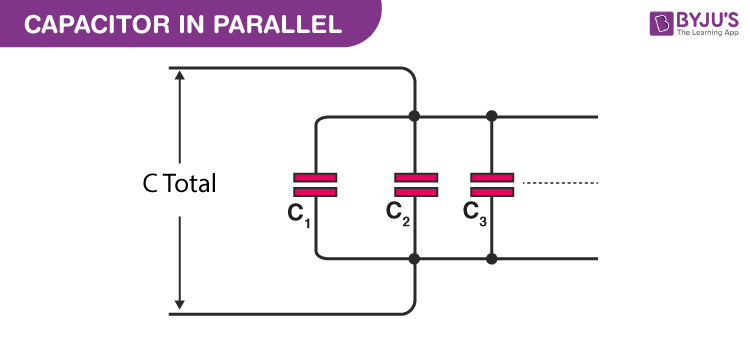# Capacitors in Parallel

We know that multiple connections of capacitors act as a single equivalent capacitor. The total capacitance of this equivalent single capacitor depends both on the individual capacitors and how they are connected. There are two simple and common types of connections: series connection and parallel connection. In this article, let us discuss in detail capacitors in parallel and the formula used to find the equivalent capacitance of the parallel combination of capacitors.

## Capacitors in Parallel

The total capacitance can be easily calculated for both series connections as well as for capacitors in parallel. Capacitors may be placed in parallel for various reasons. A few reasons why capacitors are placed in parallel are:

• Higher levels of capacitance
• To provide an exact value which otherwise may not be available
• To provide a distributed capacitance on a printed circuit board

## Capacitors In Parallel Formula

Following is the table explaining the capacitors in the parallel formula:

 Ceq=C1+C2+C3+……+CnThe total capacitance of a set of parallel capacitors is simply the sum of the capacitance values of the individual capacitors. Theoretically, there is no limit to the number of capacitors that can be connected in parallel. But certainly, there will be practical limits depending on the application, space, and other physical limitations.

You may also want to check out the following links:

The below video explains the parallel combination of capacitors:### Applications of Parallel Capacitors

By combining several capacitors in parallel, the resultant circuit will be able to store more energy as the equivalent capacitance is the sum of individual capacitances of all capacitors involved. This effect is used in the following applications.

• DC power supplies sometimes use parallel capacitors in order to better filter the output signal and eliminate the AC ripple.
• Energy storage capacitor banks are used for power factor correction with inductive loads.
• Capacitive storage banks are used in the automotive industry for regenerative braking in large vehicles such as trams and hybrid cars.

Using capacitors in parallel provides additional flexibility in their use.

#### See the video below to learn important JEE questions on electrostatic potential and capacitance.## Frequently Asked Questions – FAQs

### What is the formula to find the equivalent capacitance in parallel combinations?

Ceq=C1+C2+C3+……+Cn

### What are the two types of connections that can be achieved with a capacitor?

The following are the types of connections:
• Series connection
• Parallel connection

### What is a capacitor?

A capacitor is a passive electrical device that stores electrical energy. It has two terminals.

True.

### Define the capacitance of the capacitor.

The capacitance of a capacitor is the ratio of the magnitude of the charge on either conductor to the potential difference between the conductors forming the capacitor.
Test your knowledge on Capacitors in parallel